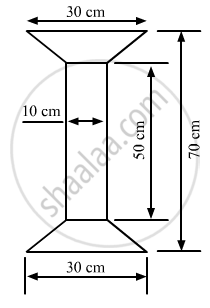Share

# Find the Area of Fig. 20.35 as the Sum of the Areas of Two Trapezium and a Rectangle. - Mathematics

Course
ConceptArea of Trapezium

#### Question

Find the area of Fig. 20.35 as the sum of the areas of two trapezium and a rectangle.#### Solution

The given figure is:In the given figure, we have a rectangle of length 50 cm and width 10 cm, and two similar trapeziums with parallel sides as 30 cm and 10 cm at both ends.

Suppose x is the perpendicular distance between the parallel sides in both the trapeziums.

We have:

Total length of the given figure = Length of the rectangle + 2 x Perpendicular distancebetween the parallel sides in both the trapeziums

$70 = 50 + 2 \times x$

$2\times x=70-50=20$

$x=\frac{20}{2}=10 cm$

Now, area of the complete figure = (area of the rectangle with sides 50 cm and 10 cm) + 2 x (area of the trapezium with parallel sides 30 cm and 10 cm, and height 10 cm

$=(50\times10)+2\times[\frac{1}{2}\times(30+10)\times(10)]$

$= 500 + 2 \times $

$= 900 {cm}^2$

Is there an error in this question or solution?

#### APPEARS IN

RD Sharma Solution for Mathematics for Class 8 by R D Sharma (2019-2020 Session) (2017 to Current)
Chapter 20: Mensuration - I (Area of a Trapezium and a Polygon)
Ex. 20.2 | Q: 9 | Page no. 23

#### Video TutorialsVIEW ALL 

Solution Find the Area of Fig. 20.35 as the Sum of the Areas of Two Trapezium and a Rectangle. Concept: Area of Trapezium.
S